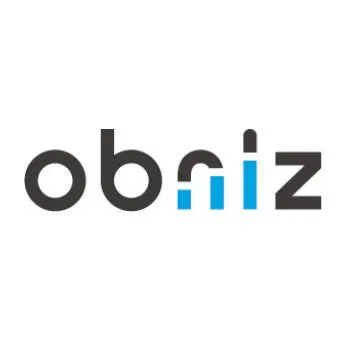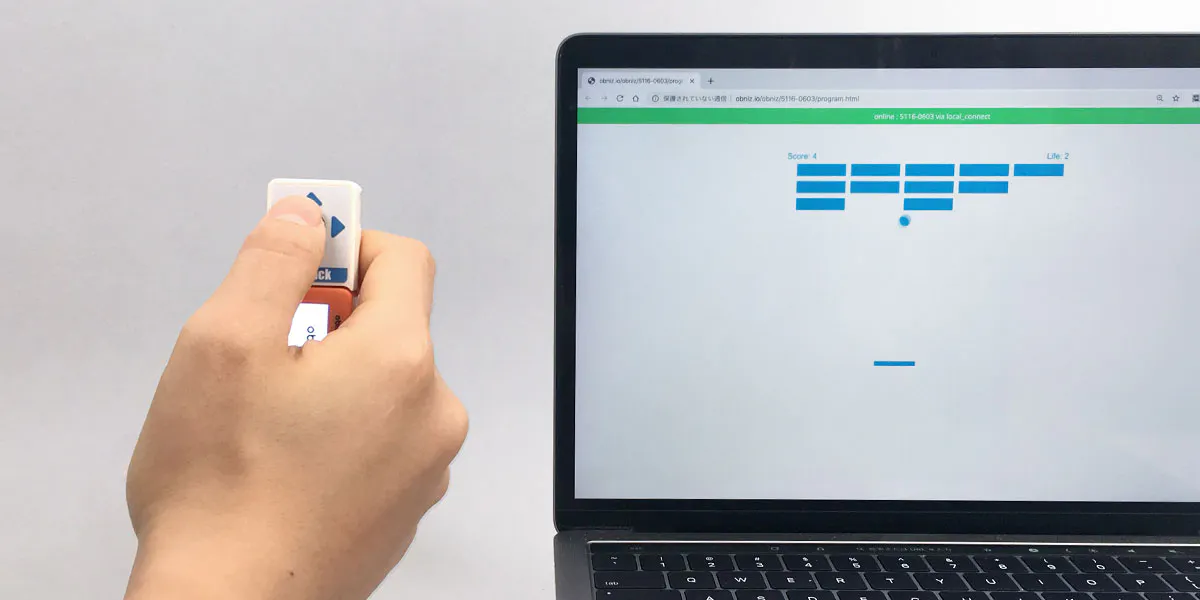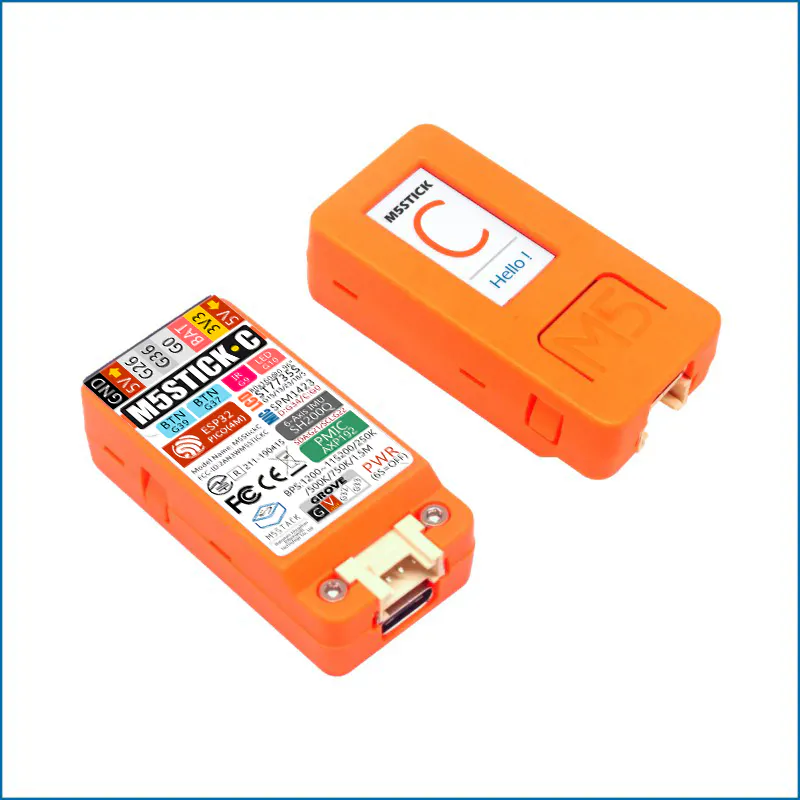Published

A simple game using M5StickC. Display the block in HTML in a web browser and control the ball with JoyStick.

IntermediateFull instructions provided2 hours320## Things used in this project

### Hardware componentsM5Stack M5StickC ESP32-PICO Mini IoT Development Board
×1
 grove joystick
×1

## Code

### Code snippet #1

Plain text
```<!DOCTYPE html>
<html>
<meta charset="utf-8" />
<title>Gamedev Canvas Workshop</title>
<style>
* { padding: 0; margin: 0; }
canvas { background: #eee; display: block; margin: 0 auto; }
</style>
<body>

<canvas id="myCanvas" width="480" height="320"></canvas>

<script>
var canvas = document.getElementById("myCanvas");
var ctx = canvas.getContext("2d");

ctx.beginPath();
ctx.rect(20, 40, 50, 50);
ctx.fillStyle = "#FF0000";
ctx.fill();
ctx.closePath();
</script>

</body>
</html>
```

### Untitled file

HTML
```<!DOCTYPE html>
<html>
<meta charset="utf-8" />
<meta name="viewport" content="width=device-width, initial-scale=1" />
rel="stylesheet"
href="https://stackpath.bootstrapcdn.com/bootstrap/4.3.1/css/bootstrap.min.css"
/>
<script src="https://obniz.io/js/jquery-3.2.1.min.js"></script>
<script
src="https://unpkg.com/obniz@3.4.0/obniz.js"
crossorigin="anonymous"
></script>
<script src="https://unpkg.com/m5stickcjs/m5stickc.js"></script>
<style>
#container {
width: 600px;
margin: 50px auto auto auto;
}
</style>
<body>
<div id="obniz-debug"></div>
<div id="container">
<canvas id="canvas" width="600" height="450"></canvas>
</div>
<script>
const canvas = document.getElementById("canvas");
const ctx = canvas.getContext("2d");
const fps = 1000 / 50;
let run = true;
let x = canvas.width / 2;
let y = canvas.height - 30;
let dx = 4;
let dy = -4;
let boardHeight = 10;
let boardWidth = 85;
let boardX = (canvas.width - boardWidth) / 2;
let blockRowCount = 5;
let blockColumnCount = 3;
let blockWidth = canvas.width / blockRowCount - 20;
let blockHeight = 25;
let blockOffsetTop = 30;
let blockOffsetLeft = 30;
let score = 0;
let life = 3;
let blocks = [];
function Block() {
this.x = 0;
this.y = 0;
this.alive = true;
}
for (let c = 0; c < blockColumnCount; c++) {
blocks[c] = [];
for (let r = 0; r < blockRowCount; r++) {
blocks[c][r] = new Block();
}
}
function collisionDetection() {
for (let c = 0; c < blockColumnCount; c++) {
for (let r = 0; r < blockRowCount; r++) {
let b = blocks[c][r];
if (b.alive) {
if (
x > b.x &&
x < b.x + blockWidth &&
y > b.y &&
y < b.y + blockHeight
) {
dy = -dy;
b.alive = false;
score++;
if (score == blockRowCount * blockColumnCount) {
run = false;
ctx.font = "40px Arial";
ctx.fillStyle = "#00FF3B";
ctx.fillText("CLEAR!!", canvas.width / 3, canvas.height / 2 - 20);
}
}
}
}
}
}
function frameOutDetection() {
if (x + dx > canvas.width - ballRadius || x + dx < ballRadius) {
dx = -dx;
}
if (y + dy < ballRadius) {
dy = -dy;
} else if (y + dy > canvas.height - ballRadius) {
if (x > boardX && x < boardX + boardWidth) {
dy = -dy;
} else {
life--;
if (!life) {
run = false;
ctx.font = "40px Arial";
ctx.fillStyle = "#FF1111";
ctx.fillText("GAME OVER", canvas.width / 3, canvas.height / 2 - 20);
} else {
x = canvas.width / 2;
y = canvas.height - 30;
dx = 5;
dy = -5;
boardX = (canvas.width - boardWidth) / 2;
}
}
}
}
function drawBall() {
ctx.beginPath();
ctx.arc(x, y, ballRadius, 0, Math.PI * 2);
ctx.fillStyle = "#00A4E3";
ctx.fill();
ctx.closePath();
}
function drawBoard() {
ctx.beginPath();
ctx.rect(boardX, canvas.height - boardHeight, boardWidth, boardHeight);
ctx.fillStyle = "#00A4E3";
ctx.fill();
ctx.closePath();
}
function drawblocks() {
for (let c = 0; c < blockColumnCount; c++) {
for (let r = 0; r < blockRowCount; r++) {
if (blocks[c][r].alive) {
let blockX = r * (blockWidth + blockPadding) + blockOffsetLeft;
let blockY = c * (blockHeight + blockPadding) + blockOffsetTop;
blocks[c][r].x = blockX;
blocks[c][r].y = blockY;
ctx.beginPath();
ctx.rect(blockX, blockY, blockWidth, blockHeight);
ctx.fillStyle = "#00A4E3";
ctx.fill();
ctx.closePath();
}
}
}
}
ctx.font = "16px Arial";
ctx.fillStyle = "#00A4E3";
ctx.fillText("Score: " + score, 10, 20);
ctx.fillText("Life: " + life, canvas.width - 65, 20);
}

const obniz = new Obniz.M5StickC("OBNIZ_ID_HERE");
obniz.onconnect = async function() {
const joystick = obniz.wired("M5StickC_JoyStick", { scl: 26, sda: 0 });

let direction = 0;
async function getXLoop() {
direction = await joystick.getXWait();
await obniz.wait(10);
}

async function main() {
getXLoop();
ctx.clearRect(0, 0, canvas.width, canvas.height);
collisionDetection();
frameOutDetection();
drawblocks();
drawBall();
drawBoard();
if (direction >= 1 && boardX < canvas.width - boardWidth) {
boardX += 7;
} else if (direction <= -1 && boardX > 0) {
boardX -= 7;
}
x += dx;
y += dy;
if (run) {
setTimeout(main, fps);
}
}

main();
};

</script>
</body>
</html>
```

## Credits

### obniz developer team

3 projects • 26 followers
Development board "obniz" is controlled via the internet.